# TEXTJOIN Function

Joins text from two or more strings together with a given delimiter

## What is the TEXTJOIN Function?

The TEXTJOIN Function joins text from two or more strings together with a delimiter separating each value. Introduced in MS Excel 2016, TEXTJOIN is categorized as an Excel String/Text function.

### Formula

=TEXTJOIN(delimiter, ignore_empty, text1, [text2], …text_n)

The TEXTJOIN function uses the following arguments:

1. Delimiter (required argument) – The string that is inserted between each text value in the resulting string. The most common delimiters used are a comma or a space character.
2. Ignore_empty (required argument) – This argument helps in determining whether empty cells are included in the resulting string. If the argument is TRUE, it will ignore empty values. If it is FALSE, it will include the empty values in the results.
3. Text1, text2,….text_n – The strings that we wish to join together. The TEXTJOIN function allows us to join up to 252 strings together.

### How to use the TEXTJOIN Function in Excel?

Let’s see a few examples to understand how this function works.

#### Example 1

In this example, let’s assume we extracted information from an address and the information is received in the following manner: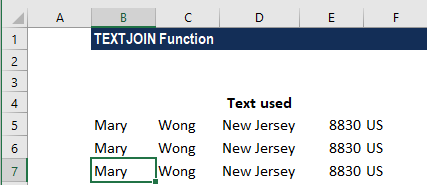Using the formula =TEXTJOIN(“, “,TRUE, B5, C5, D5, E5,F5), we can join the text strings as shown below: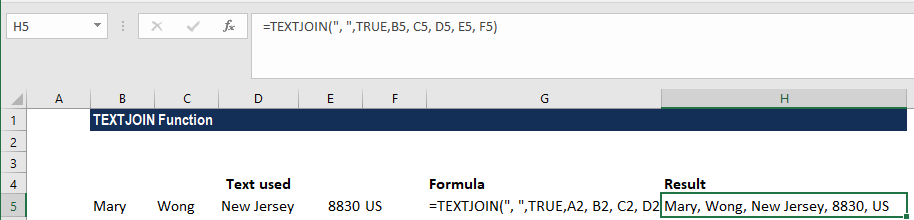Now let’s assume that we wish to add the text, “Country”, before US. In such a scenario, the formula would change to =TEXTJOIN(” “,TRUE,B6,C6,D6,E6,”Country”,F6).

Using this formula, we will get the result below: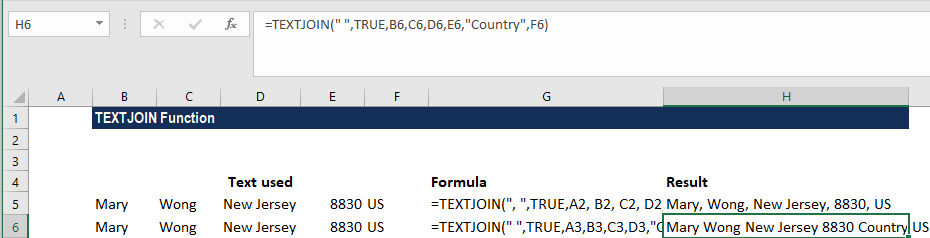Now suppose we wish to put a space as a delimiter. In such a scenario, the formula to use will be =TEXTJOIN(” “,TRUE,B7, C7, D7, E7,F7).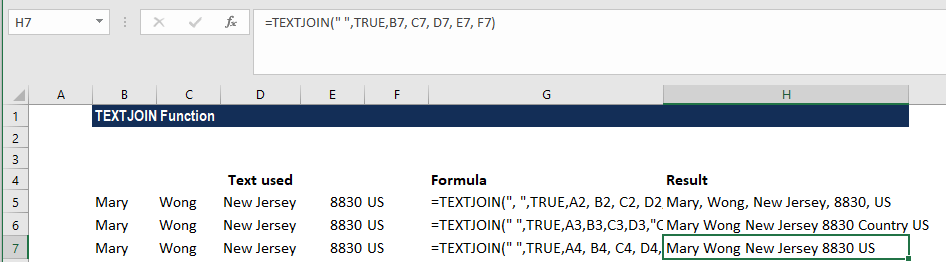#### Example 2

Let’s see an example of how to join more than two columns (using the data in columns below).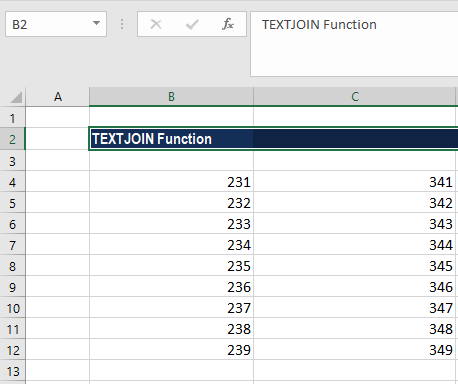If we use the formula =TEXTJOIN(“, “, TRUE, B4:C12) then Excel will give the following result: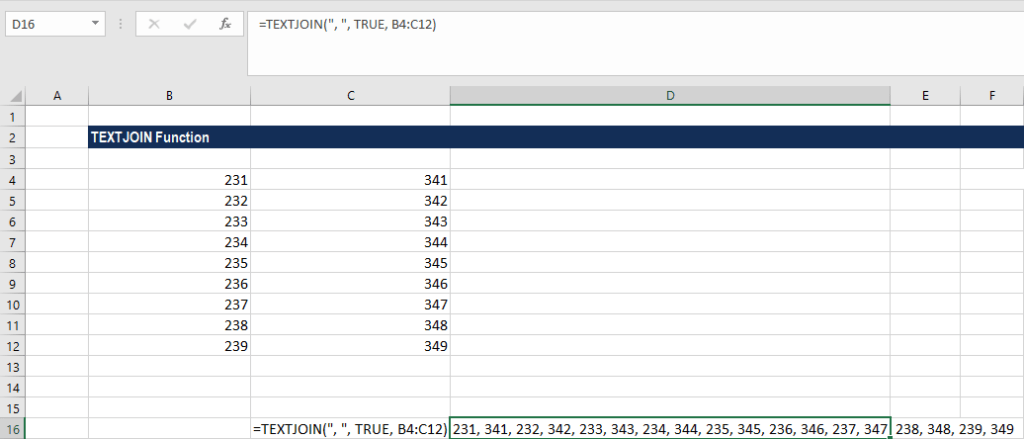#### Example 3

Let’s take an example where we are given the name, surname, and date of anniversary in separate columns and we wish to join them. In this scenario, we need to remember that if we directly enter the date, Excel will return a number, as Excel store dates in number format.

Using the date below: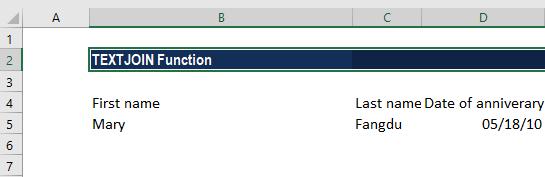If for this data we enter the formula =TEXTJOIN( ” “, TRUE, B5:D5,)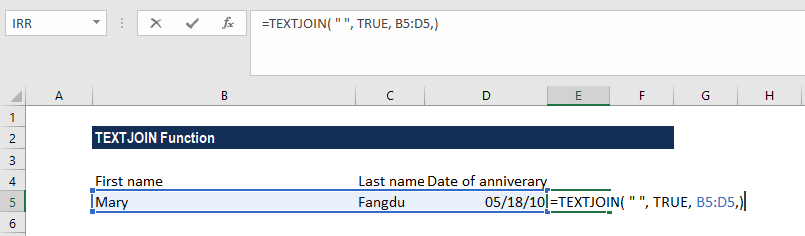Excel will give the result below: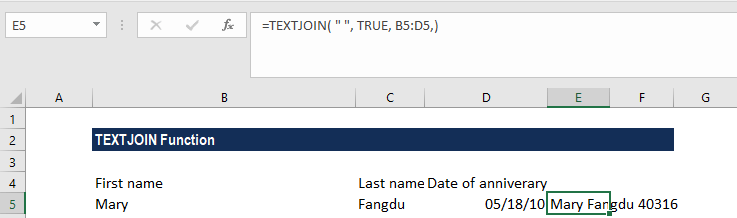Hence, we need to convert the date into text string first. So, the formula that we need to use would be =TEXTJOIN( ” “, TRUE, B5:D5, TEXT(C2, “mm/dd/yyyy”) ). We get the result below: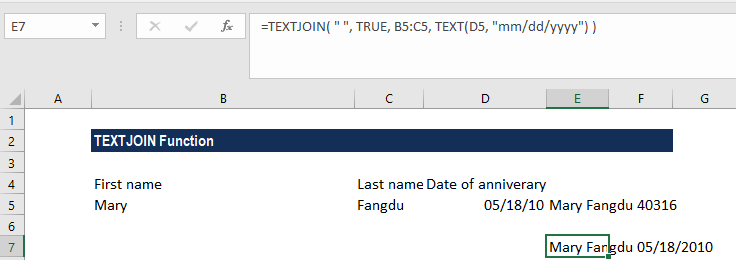#### More on the TEXTJOIN Function

1. #VALUE! error – Excel will return this error when the resulting string exceeds the cell limit, which is 32767 characters.
2. The function can handle 252 text arguments.
3. This function is quite similar to the CONCAT function. The difference between the two is that while TEXTJOIN accepts a delimiter, CONCAT doesn’t.
4. #NAME? error – Occurs when you use an older version of Excel that doesn’t support this function.
5. #NULL! error – Occurs when we forget to put the comma between the strings we wish to combine.
6. It gives the user the flexibility to use cell ranges rather than individual cell references.

Thanks for reading CFI’s guide to important Excel functions! By taking the time to learn and master these functions, you’ll significantly speed up your financial analysis. To learn more, check out these additional CFI resources:

• Excel Functions for Finance